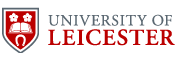informatics

Abstract

A programming metalogic is a formal system into which programming languages can be translated and given meaning. The translation should both reflect the structure of the language and make it easy to prove properties of programs. This thesis develops certain metalogics using techniques of category theory and treats recursion in a new way.

The notion of a category with fixpoint object is defined. Corresponding to this categorical structure there are type theoretic equational rules which will be present in all of the metalogics considered. These rules define the fixpoint type which will allow the interpretation of recursive declarations. With these core notions FIX categories are defined. These are the categorical equivalent of an equational logic which can be viewed as a very basic programming metalogic. Recursion is treated both syntactically and categorically.

The expressive power of the equational logic is increased by embedding it in an intuitionistic predicate calculus, giving rise to the FIX logic. This contains propositions about the evaluation of computations to values and an induction principle which is derived from the definition of a fixpoint object as an initial algebra. The categorical structure which accompanies the FIX logic is defined, called a FIX hyperdoctrine, and certain existence and disjunction properties of FIX are stated. A particular FIX hyperdoctrine is constructed and used in the proof of the above properties.

PCF-style languages are translated into the FIX logic and computational adequacy results are proved. Two languages are studied: Both are similar to PCF except one has call by value recursive function declarations and the other higher order conditionals.

A dependently typed equational logic containing a fixpoint type and a universal type is given together with its related categorical structure, namely a FIX category with attributes. A representation theorem for Scott predomains is proved, which gives rise to a concrete example of such a FIX category with attributes. Recursive domain equations give rise to endofunctions on the universal type; using the fixpoint type we may solve for fixpoints of such endofunctions and thus obtain a solution of the original domain equation as the type coded by the fixpoint.

Other

My Ph.D. Thesis is available as the University of Cambridge Computer Laboratory Technical Report Number 247. My Ph.D. examiners were

Professor J.M.E. Hyland, Department of Pure Mathematics and Mathematical Statistics, Cambridge, England

and

Prof. E. Moggi, Dip. di Matematica, Univ. di Genova, Italy.

 Author: Roy Crole.© University of Leicester March 2013. Last modified: 12th April 2017, 10:39:59Informatics Web Maintainer. This document has been approved by the Head of Department.